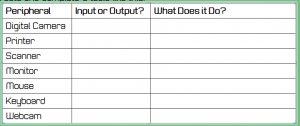## Lesson Aims

• Understand Algorithms
• Explain what they do and correct and complete them

Quiz

## Main

Algorithms presentation

Algorithm notes

Plenary

Exam question

Complete the question for homework next lesson

## Introduction to Computer Hardware

#### Lesson Aim

• Understand the differences between input and output devices and recognise where sensors are used in everyday objects

#### Starter

As a class view presentation and identify the key computer peripherals as input, output or storage devices.

Presentation

#### Main

Create a Word Document with the title Inputs and Outputs.

Create and complete a table like this:For each of the different types of sensor, state an everyday item that uses this sensor and explain how it is used. Here’s a reminder of the different sensors:

• Light
• Temperature
• Moisture
• Pressure
• Motion
• Smoke

#### Plenary

1. What is a peripheral device?
1. Give an example of an output device connected to a computer.
1. Is a mouse an input or an output?
1. Give an example of an everyday device that contains a sensor. How is it used?

## Lesson Aim

• Explain the function of a CPU
• Explain function of different parts of the CPU

## Introduction

CPU Presentation

Complete  the worksheet below: CPU Worksheet

## Lesson Aim

• Explain the functions of an output device
• Identify the main output devices

You need to produce a powerpoint presentation about output devices. Your presentation will need a title slide and then a slide for each output device.

Each slide needs  the following

• Title
• Image of the output device
• A couple of pieces of information about the device

You may use the internet to get images and information, but do not copy and paste text.

## Lesson Aim

• Understand the need for an algorithm
• Explain what pseudocode is
• Explain what a flowchart is

Theory

Algorithms presentation template